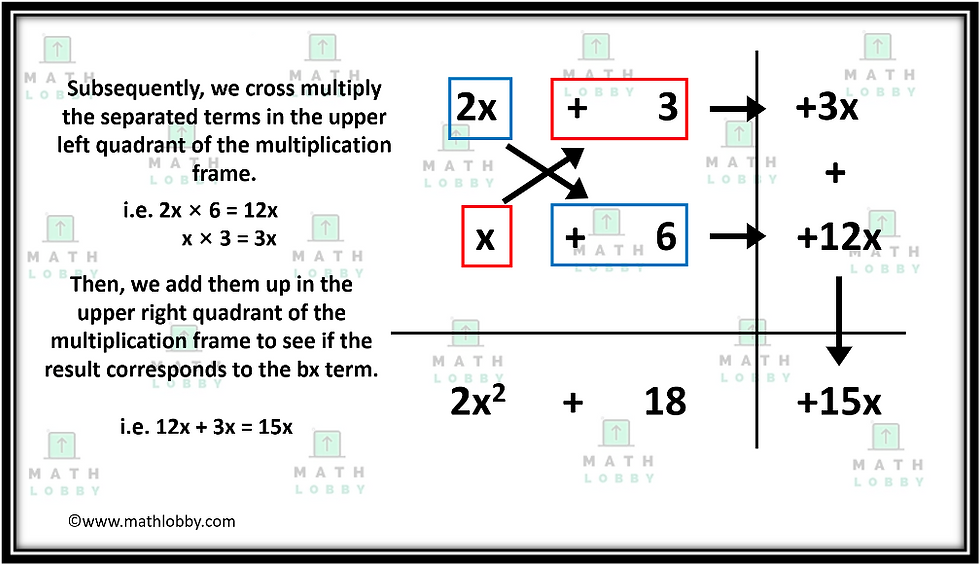top of page
Search

# How to factorize quadratic equations of the form ax^2 + bx + c, where a ≠ 1

In this note, you will learn:

· How to factorize quadratic equations of the form ax^2 + bx + c, (where a ≠ 1)

Previously, we discussed on how to factorize quadratic equations in the form of x^2 + bx + c, where c > 0 ( and b < 0). For those who missed it, be sure to check it out from the link above!

Today, we will be dealing with another version of quadratic equations which is the factorization of quadratic equations of the form ax^2 + bx + c, where a ≠ 1.

Like the previous article, we are going to apply the guess and check together with logic and reasoning to factorize the problem in hand. However, we will have to be careful since there are more possible pairs of combinations to consider! How so you may ask? Let’s try a practice question for you to visualize.

## How to factorize quadratic equations of the form ax^2 + bx + c, where a ≠ 1

For this example, let’s take 2x^2 + 15x + 18 as the problem we are trying to solve here.First step is similar to the previous case, so explanation is not required, but if you are still unsure, do refer back to the previous example!As shown in the diagram above, for the second step, there should be a sequence of thought process.

For example, if we were to take the factors of 18 as 1 and 18, we can see the it is impossible to obtain +15x since 18 > 15, and no sum will yield the desired bx term.

Then what about the factors 2 and 9?

Similarly, this option is also not viable as (2x) (2) + (x)(9) = 13x and (2x) (9) + (x)(2) = 20x. The answers obtained are either larger or smaller than our desired bx term.

Let’s see how the last option is able to help us yield our desired bx term. The factors 3 and 6 when multiplied with x and 2x is able to give us (2x) (3) + (x)(6) = 12x and (2x) (6) + (x)(3) = 15x.

From this, we are able to see that one of the combinations is able to yield our desired answer, hence this is why we chose it as our most viable option.Finally, we can cross multiply the values in the upper left quadrant and we will check if we end up with the desired bx term that we were supposed to obtain. In this case, we did it! The answer after factorizing 2x^2 + 15x + 18 is equals to (2x + 3) (x + 6)!

And that’s all for today, students! Math Lobby hopes that after this article, you have a clear understanding on the methods of how to factorize quadratic equations using the multiplication frame!

If you have any pending questions, please do go on to our Facebook page, Instagram or contact us directly at Math Lobby! We have certified mathematics tutors to aid you in your journey to becoming a better student!

As always: Work hard, stay motivated and we wish all students a successful and enjoyable journey with Math Lobby!

Visit and FOLLOW our Instagram page at https://www.instagram.com/mathlobbymotivation/

*

*

*